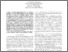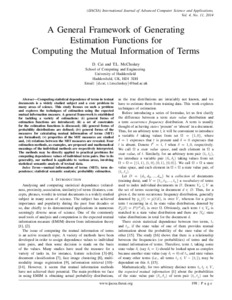Cai, Di and McCluskey, T.L. (2014) A General Framework of Generating Estimation Functions for Computing the Mutual Information of Terms. International Journal of Advanced Computer Science and Applications, 4 (11). pp. 198-208. ISSN 2158-107X
Abstract

Computing statistical dependence of terms in textual documents is a widely studied subject and a core problem in many areas of science. This study focuses on such a problem and explores the techniques of estimation using the expected mutual information measure. A general framework is established for tackling a variety of estimations: (i) general forms of estimation functions are introduced; (ii) a set of constraints for the estimation functions is discussed; (iii) general forms of probability distributions are defined; (iv) general forms of the measures for calculating mutual information of terms (MIT) are formalised; (v) properties of the MIT measures are studied and, (vi) relations between the MIT measures are revealed. Four estimation methods, as examples, are proposed and mathematical meanings of the individual methods are respectively interpreted. The methods may be directly applied to practical problems for computing dependence values of individual term pairs. Due to its generality, our method is applicable to various areas, involving statistical semantic analysis of textual data

Information
Library
DocumentsPreview
Paper_28-A_General_Framework_of_Generating.pdf - Published Version